Pythagoras Theorem: Baravalle demonstration

We can play with two previous interactive applications to 'see' demonstrations of the Pythagorean Theorem. One is inspired in Euclid (although Euclid did not have a dynamic approach) and the other uses a tiling.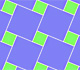We can see Pythagoras' Theorem in a tiling. It is a graphic demonstration of Pythagoras' Theorem we can see in some floor made using squares of two different sizes.

The Pythagorean Theorem (or Theorem of Pythagoras) is one of the most famous theorems of Mathematics. It is a property of right-angled triangles.

Then the Theorem of Pythagoras states that the square on the hypotenuse equals the sum of the (areas of the) squares on the other two sides.In this page we can interact with another dynamic and interactive demonstration of the Pythagorean Theorem devised by Hermann Baravalle (1945).

The main idea is that if a parallelogram is changed by a shearing (preserving its base and altitude), its area remains constant.And if we consider a movement (a translation in this case) of a parallelogram the area does not change.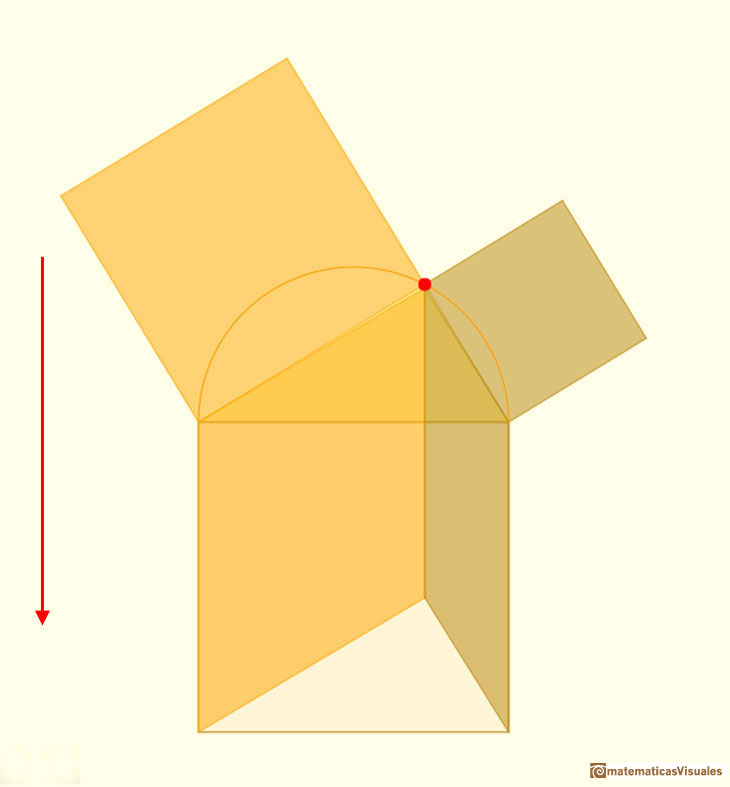Another shearing: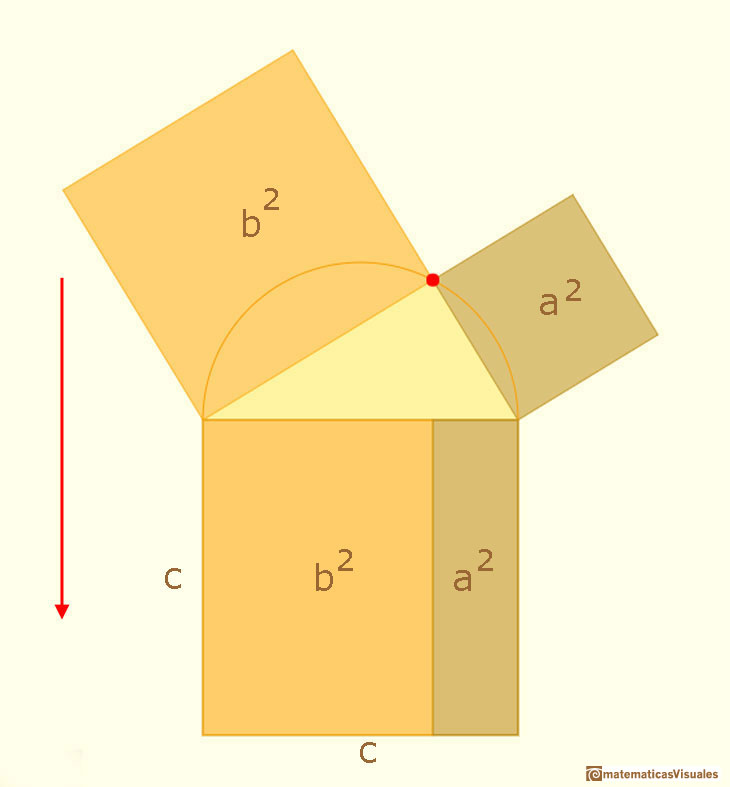This is a demonstration of the Pythagorean Theorem:

REFERENCES

Euclides, The Elements
Alexander Bogomolny, Cut the Knot. Pythagorean theorem.
H.S.M. Coxeter, 'Introduction to Geometry', John Wiley and Sons, Second edition, pp. 8-9.
John Stillwell, "Mathematics and its History", Springer-Verlag, New York, 2002.
Martin Gardner, 'Sixth Book of Mathematical Diversions from "Scientific American"'. Scribner, 1975.
Eli Maor, "The Pythagorean theorem: a 4000-year history", Princeton University Press, United States of America, 2007.
F.J. Swetz and T.I. Kao, "Was Pythagoras chinese?", The Pennsylvania State University Press, United States of America, 1977.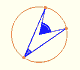Central angle in a circle is twice the angle inscribed in the circle.We want to calculate the surface area of sections of a sphere using the Pythagorean Theorem. We also study the relation with the Geometric Mean and the Right Triangle Altitude Theorem.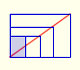There is a standarization of the size of the paper that is called DIN A. Successive paper sizes in the series A1, A2, A3, A4, and so forth, are defined by halving the preceding paper size along the larger dimension.You can draw a regular pentagon given one of its sides constructing the golden ratio with ruler and compass.Using Cavalieri's Principle we can calculate the volume of a sphere.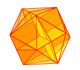The twelve vertices of an icosahedron lie in three golden rectangles. Then we can calculate the volume of an icosahedronThe volume of a tetrahedron is one third of the prism that contains it.The volume of an octahedron is four times the volume of a tetrahedron. It is easy to calculate and then we can get the volume of a tetrahedron.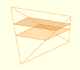In his article 'Two Surprising Theorems on Cavalieri Congruence' Howard Eves describes an interesting tetrahedron. In this page we calculate its cross-section areas and its volume.Howard Eves's tetrahedron is Cavalieri congruent with a given sphere. You can see that corresponding sections have the same area. Then the volumen of the sphere is the same as the volume of the tetrahedron. And we know how to calculate this volumen.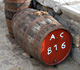Studying the volume of a barrel, Kepler solved a problem about maxima in 1615.